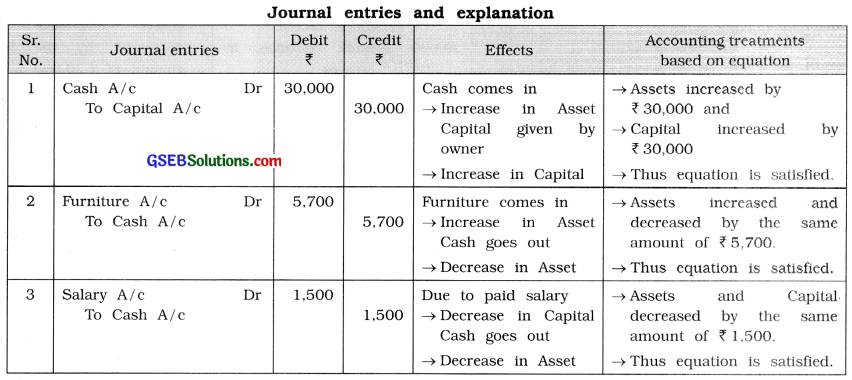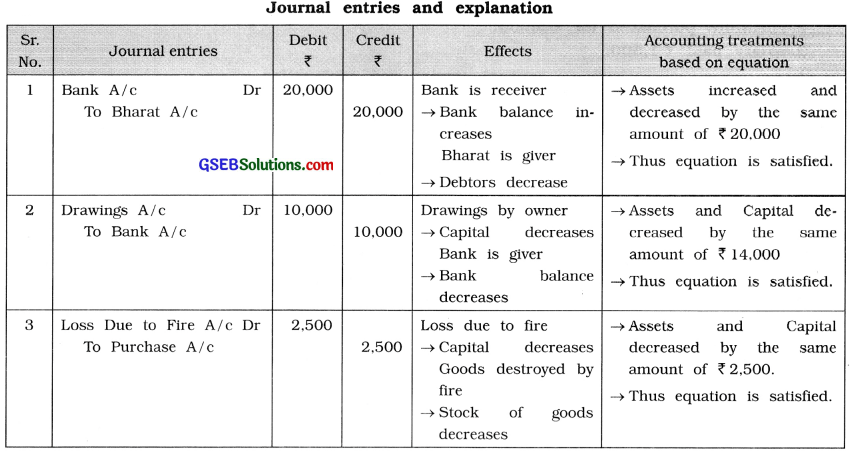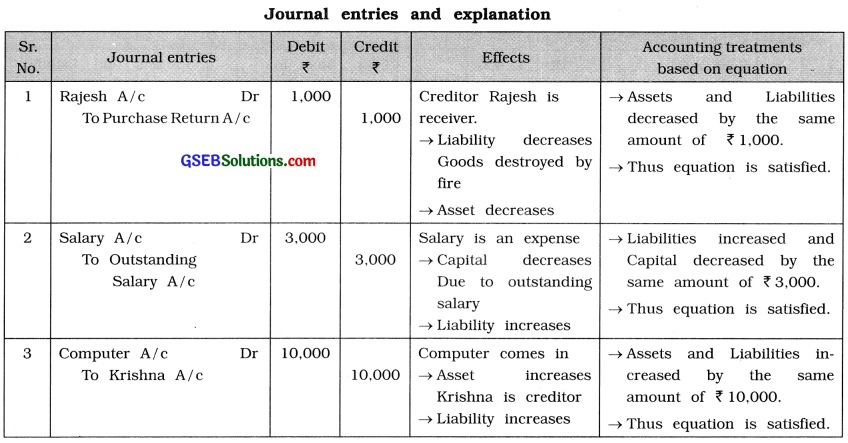# GSEB Class 11 Accounts Important Questions Part 1 Chapter 5 Accounting Equation and Business Transactions

Gujarat Board GSEB Class 11 Commerce Accounts Important Questions Part 1 Chapter 5 Accounting Equation and Business Transactions Important Questions and Answers.

## GSEB Class 11 Accounts Important Questions Part 1 Chapter 5 Accounting Equation and Business Transactions

Very Short Questions (VSQs)
Answer the following questions in one sentence : [1 mark each ]

Question 1.
Goods of ₹ 3,000 is purchased on cash. Explain accounting treatment based on equation for this transaction.
Goods of ₹ 3,000 is purchased on cash.
Effects :
(i) Goods come in → Increase in Asset
(ii) Cash goes out → Decrease in Asset

Conclusion:
→ Assets have increased and decreased by the same amount of ₹ 3,000.
→ Thus, equation is satisfied.

Question 2.
Opened a bank account by depositing cash ₹ 10,000. Explain accounting treatment based on equation for this transaction.
Effects :
(i) Bank is receiver → Bank balance (Asset) increases
(ii) Cash goes out → Cash (Asset) decreases

Conclusion :
→ Assets have increased and decreased by the same amount of ₹ 10,000.
→ Thus, equation is satisfied.Question 3.
Received dividend of ₹ 1,200 by cheque. Explain accounting treatment based on equation for this transaction.
Effects :
(i) Bank is receiver → Bank balance (Asset) increases
(ii) Dividend is an income → Capital increases

Conclusion :
→ Assets and Capital have increased by the same amount of ₹ 1,200.
→ Thus, equation is satisfied.

Question 4.
Calculate the amount of cash, if total assets (except cash) = ₹ 60,000; total liabilities = ₹ 50,000 and capital = ₹ 40,000.
Accounting equation is A = C + L
∴ Cash + Other Assets = Capital + Liabilities
∴ Cash + 60,000 = 40,000 + 50,000
∴ Cash + 60,000 = 90,000
∴ Cash = 90,000-60,000 = 30,000
∴ Amount of cash is ₹ 30,000.

Question 5.
In the company form, what is prepared instead of Trading Account and Profit and Loss Account ?
In the company form, Income Statement is prepared instead of Trading Account and Profit and Loss Account.

Question 6.
According to the terminology of accounting how are known as two components of Balance Sheet?
According to the terminology of accounting, two components of balance are known as Capital and Liability side and Assets side.

Question 7.
Explain accounting equation.
A = C + L is the accounting equation; where A = Assets, C = Capital and L = Liabilities.

Question 8.
In accounting equation, for which thing is directly effected on Balance Sheet?
In accounting equation, effect of business transaction is come on Balance Sheet.Question 9.
When capital introduced by owner in business, which effect is created on accounting equation ?
When capital introduced by owner in business, Assets (A) and Capital (C) have increased by the same amount.

Question 10.
How many components of annual accounts are there? Which are they?
There are three components of annual accounts :

2. Profit and Loss account and
3. Balance Sheet.

Question 11.
What is disclosed Balance Sheet?
Balance Sheet is disclosed financial status of business.

Question 12.
Under Double Entry System, where the balance of trading account is transferred?
Under Double Entry System, the balance of trading account is transferred to Profit and Loss account.

Question 13.
Explain effect on capital, when sales is made with profit or with loss.
When sales is made with profit, capital would increase and sales is made with loss, capital would reduce.

Question 14.
Borrowed loan from Dena Bank. For this transaction, show how the accounting equation A = C + L is satisfied.
Borrowed loan from Dena Bank. Here Assets (A) and Liabilities increase by the same amount thereby satisfying the accounting equation A = C + L.Question 15.
Which type of approach is A = C + L ?
A = C + L is a modern approach.

Question 16.
State the meaning of accounting equation.
Equation which signifies that the assets of a business are always equal to the total of its liabilities and capital is called accounting equation.

Question 17.
Which rules are used for preparing books of accounts?
Under traditional approach, rules of personal accounts, real accounts and nominal accounts are used for preparing books of accounts.

Question 18.
When equation method can be understood easily ?
Equation method can be understood easily, if business transactions are classified as under:

1. Transaction related to goods,
2. Transaction related to revenues and expenses,
3. Transaction related to assets and
4. Transaction related to borrowing/lending of funds.

Question 19.
On the which basis of annual accounts are prepared?
Annual accounts are prepared on the basis of Journal – Subsidiary books – Posting-Trial Balance and Adjustments.

Question 20.
Goods were stolen away from business. Explain conclusion based on accounting equation for this transaction.
Capital amount decreases due to loss by theft and with the same amount assets (stock of goods) also decreases due to goods stolen away.Question 21.
When cash or goods withdrawn made by owner from business, which effect are made on capital and on liability?
When cash or goods withdrawn made by owner from business, capital and liability decrease by the same amount.

Question 22.
Find out the value of capital, if liabilities = ₹ 80,000 and Assets = ₹ 1,20,000.
Assets = Capital + Liabilities
∴ Capital = Assets – Liabilities
= 1,20,000 – 80,000
= 40,000
∴ Value of Capital = ₹ 40,000

Question 23.
Which financial statement displays the revenues and expenses of a company for a period of time?
Income Statement displays the revenues and expenses of a company for a period of time.

Question 24.
Which financial statement uses the expanded accounting equation?
Balance Sheet uses the expanded accounting equation.

Question 25.
What is the significant objective of financial accounting ?
The significant objective of financial accounting is to prepare financial accounts.

Question 26.
At the end of the year, where the balance of Profit and Loss account is transfered ?
At the end of the year, balance of Profit and Loss account is transferred to Capital account in Balance Sheet.Question 27.
Explain two important components of Balance Sheet.
There are two important components of Balance Sheet:

1. Capital and Liabilities and
2. Assets.

Question 28.
As per the norms of Double Entry System, how is the total of two components of Balance Sheet?
As per the norms of Double Entry System, the total of two components of Balance Sheet is always equal / identical.

Question 29.
Which financial statement is closely related to the accounting equation?
The closely related financial statement to accounting equation is the Balance Sheet.

Short Sums for Accounting Equation
Write journal entries from the given transactions and explain accounting treatments based on equation : [3 marks each]

Question 1.
Write journal entries for the following transactions and explain accounting treatments based on equation :
1. Brought ₹ 30,000 and commenced business.
2. Furniture purchased for ₹ 5,700.
3. Salary paid ₹ 1,500.Question 2.
Write journal entries for the following transactions and explain accounting treatments based on equation :
1. Borrowed a loan of ₹ 35,000 from a friend Shripal.
2. Sold goods of ₹ 20,000 for ₹ 26,000 to Jaydeep.
3. Received commission of ₹ 3,000 in cash.Question 3.
Write journal entries for the following transactions and explain accounting treatment based on equation:
1. Customer Bharat gave ₹ 20,000, which is deposited in bank.
2. Paid life insurance premium of ₹ 10,000 by cheque.
3. Goods of ₹ 2,500 destroyed by fire.Question 4.
Write journal entries for the following transactions and explain accounting treatments based on equation:
1. Goods of ₹ 1,000 returned to Rajesh.
2. Salary outstanding is ₹ 3,000.
3. A computer of ₹ 10,000 is purchased from Krishna.Multiple Choice Questions (MCQs)
Write the correct option from those given below each question : [1 mark each]

Question 1.
When goods distributed as free sample …………….
(a) decrease in asset – decrease in liability.
(b) increase in asset – increase in liability.
(c) increase in asset – increase in capital.
(d) decrease in asset – decrease in capital.
(d) decrease in asset – decrease in capital.

Question 2.
When revenue expenses are paid in cash ……………….
(a) increase in asset – increase in liability.
(b) increase in asset – increase in capital.
(c) decrease in asset – decrease in capital.
(d) decrease in asset – decrease in liability.
(c) decrease in asset – decrease in capital.

Question 3.
At the end of year, after the closing of Profit & Loss account, where will be balance of it transfered ?
(b) To Bank A/c
(c) To Suspense A/c
(d) To Balance sheet
(d) To Balance sheet

Question 4.
…………………. method is A = C + L for presentation
of accounting transactions.
(a) American
(b) Indian
(c) Japanese
(d) Chinese
(a) American

Question 5.
(a) decrease in asset – decrease in capital.
(b) increase in asset – increase in capital.
(c) decrease in asset – decrease in liability.
(d) decrease in asset – increase in liability.
(a) decrease in asset – decrease in capital.

Question 6.
When goods purchased in cash ………………
(a) increase in asset – increase in capital.
(b) one would increase – another would decrease.
(c) increase in asset – increase in liability.
(d) increase in capital – decrease in liability.
(b) one would increase – another would decrease.Question 7.
When funds borrowed …………………
(a) increase in asset – increase in liability.
(b) one would increase – another would decrease.
(c) increase in asset – increase in capital.
(d) increase in capital – decrease in liability.
(a) increase in asset – increase in liability.

Question 8.
When asset purchased in cash ……………..
(a) increase in asset – increase in liability.
(b) one would double – another would double.
(c) one would increase – another would decrease.
(d) increase in capital – decrease in liability.
(c) one would increase – another would decrease.

Question 9.
When goods destroyed by fire ……………
(a) one would decrease – another would increase.
(b) one would decrease – another would decrease.
(c) decrease in asset – decrease in liability.
(d) decrease in asset – decrease in capital.
(d) decrease in asset – decrease in capital.

Question 10.
When asset of business sold on credit ……………..
(a) one would increase – another would decrease.
(b) increase in liability – decrease in liability.
(c) increase in asset – increase in capital.
(d) decrease in capital – increase in asset.
(a) one would increase – another would decrease.

Question 11.
When asset pruchased on credit ……………….
(a) increase in asset – increase in capital.
(b) increase in asset – increase in liability.
(c) increase in asset – decrease in capital.
(d) one would increase – another would decrease.
(b) increase in asset – increase in liability.

Question 12.
When revenue income outstanding ……………..
(a) one would decrease – another would increase.
(b) increase in asset – increase in liability.
(c) increase in asset – increase in capital.
(d) increase in liability – decrease in asset.
(c) increase in asset – increase in capital.Question 13.
The basic accounting equation is ……………..
(a) Assets = Expenses + Incomes
(b) Assets = Capital + Liabilities
(c) Assets = Cash + Capital
(d) Assets = All expenses + Capital
(b) Assets = Capital + Liabilities

Question 14.
At the end of year, after closing the Trading account, where will be it transfered ?
(a) To Capital A/c
(b) To Profit & Loss A/c
(c)To Balance Sheet
(d) To Suspense A/c
(b) To Profit & Loss A/c

Question 15.
At the end of year, after the closing of Capital account, where will be it transfered ?
(b) To Profit & Loss A/c
(c) To Balance Sheet
(d) To Drawings A/c
(c) To Balance Sheet

Question 16.
Under Double Entry Accounting System, sum of all assets is always …………….
(a) equal to sum of all liabilities.
(b) equal to sum of capital.
(c) equal to sum of capital and liabilities.
(d) equal to difference of capital and liabilities.
(c) equal to sum of capital and liabilities.

Question 17.
Under Double Entry Accounting System, sum of liabilities and capital is always equal to total amount of ………………..
(a) assets
(b) receivables
(c) payables
(d) debts
(a) assets

Question 18.
How many important components of Balance Sheet are there ?
(a) Two
(b) Three
(c) Four
(d) So many
(a) TwoQuestion 19.
Which are two important components of Balance Sheet ?
(a) Capital and Liability
(b) Capital and Assets
(c) Assets and Liability
(d) Capital-Liability and Assets
(d) Capital-Liability and Assets

Question 20.
When lending of funds ……………
(a) increase in one asset – decrease in another asset.
(b) one would increase – another would decrease.
(c) increase in capital – increase in asset.
(d) increase in asset – decrease in liability.
(a) increase in one asset – decrease in another asset.

Question 21.
When owner brought personal payables/ creditors in business ……………
(a) one would increase, another would decrease.
(b) asset and liability have increased by same amount.
(c) capital and asset have decreased by same amount.
(d) increase in capital, increase in liability.
(a) one would increase, another would decrease.

Question 22.
Capital increases, if ………………. increase.
(a) Expenses
(b) Drawings
(c) Revenue
(d) Interest on drawings
(c) Revenue

Question 23.
Which of the following statement is the practical implemention of the accounting equation ?
(a) Cash flow statement
(b) Income Statement
(c) Statement of changes in capital
(d) Goods statement
(b) Income Statement

Question 24.
Depreciation decreases in …………….. .
(a) Cash
(b) Liabilities
(c) Capital
(d) Income
(c) CapitalQuestion 25.
Which of these is not included as a separate item in the basic accounting equation ?
(a) Assets
(b) Revenues
(c) Liabilities
(d) Capital
(b) Revenues

Question 26.
Which one of the following is not true for accounting equation?
(a) C + L = A
(b) A – L = C
(c) A – C = L
(d) C – L = A